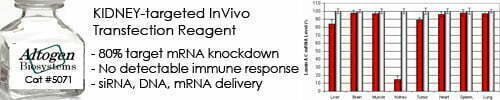••••Shop Products

# Buffer Capacity Calculator

A buffer capacity calculator is a tool that calculates the buffer capacity of a solution based on its concentration, pH, and acid dissociation constant (Ka). Buffer capacity is a measure of the ability of a buffer solution to resist changes in pH when an acid or base is added to it.

The formula for calculating buffer capacity is:

Buffer Capacity = (Concentration x Ka) / (Ka + [H+])

Where:

• Concentration is the molar concentration of the weak acid or weak base in the buffer solution
• Ka is the acid dissociation constant of the weak acid in the buffer solution
• [H+] is the concentration of hydrogen ions (protons) in the solution, which depends on the pH of the solution

To use a buffer capacity calculator, you need to input the values of concentration, Ka, and pH, and the calculator will calculate the corresponding buffer capacity for you. The resulting buffer capacity value can then be used to assess the ability of the buffer solution to resist changes in pH and to design buffer solutions for various applications in chemistry and biochemistry.

Buffer capacity is an important concept in many fields, including chemistry, biochemistry, and pharmaceuticals, where it is used to design and optimize buffer solutions for a variety of applications, such as protein purification, enzyme catalysis, and chemical synthesis.

Buffer Capacity Calculator
Amount of acid/base:
mol/liters
Initial pH:
Final pH:

Sorry, comments are closed for this post.